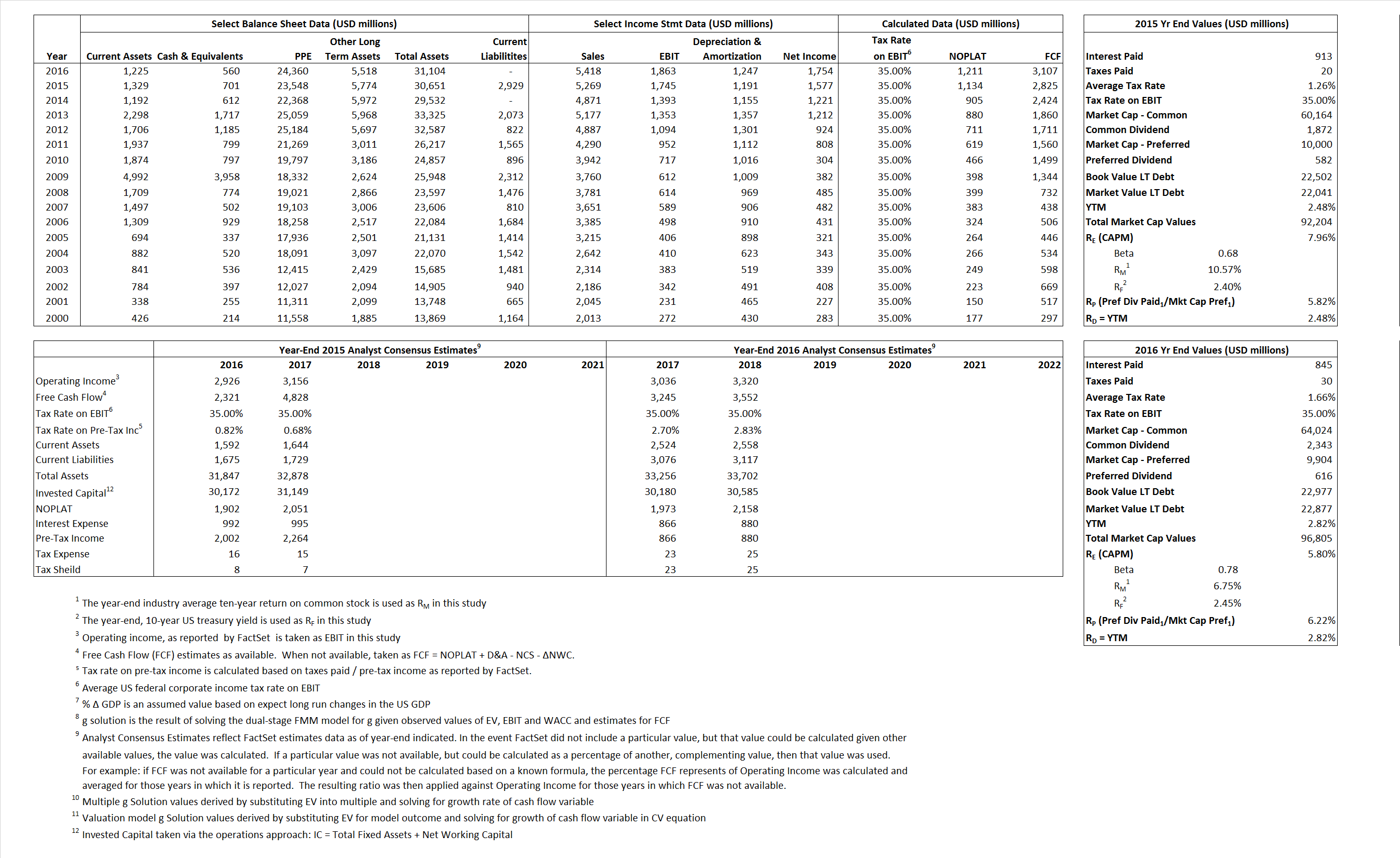# Simon Property Group Inc.

## Analyst Listing

The following analysts provide coverage for the subject firm as of May 2016:

 Broker Analyst Analyst Email Boenning & Scattergood Floris Van Dijkum fvandijkum@boenninginc.com SunTrust Robinson Humphrey Ki Bin Kim kibin.kim@suntrust.com Jefferies Omotayo Okusanya tokusanya@jefferies.com Canaccord Genuity Paul Morgan pmorgan@canaccordgenuity.com RBC Capital Markets Rich C. Moore rich.moore@rbccm.com Evercore ISI Steve Sakwa steve.sakwa@evercoreisi.com Deutsche Bank Research Vin Chao vincent.chao@db.com

## Primary Input Data## Derived Input Data

### Equational Form

Net Operating Profit Less Adjusted Taxes NOPLAT  1,134  1,211$NOPLAT\, =\, EBIT\, x\, (1 \,-\, Avg \,\,Tax\,\, Rate\,\, on\,\, EBIT)$
Free Cash Flow FCF 2,825 3,107$FCF\,=NOPLAT\,+\,Non-Cash\,Expenses-\Delta NWC\,-\,NCS$
Tax Shield TS 12 14$TS\,=\,Interest\,\,Paid\,\,x\,\, Avg \,\,Tax\,\,Rate\,\, on\,\, Pre-Tax\,\, Income$
Invested Capital IC 27,722 31,104$IC\,=\,Fixed\,\,Operating\,\,Assets\,\,+\,\,Net\,\, Working\,\, Capital$
Return on Invested Capital ROIC 4.09% 3.89%$ROIC\,=\,\frac { NOPLAT }{ IC }$
Net Investment NetInv (619) 4,629$NetInv\,=\,{ {IC}_{1}}-{{IC}_{0}}+Depreciation$
Investment Rate IR  -54.57%  382.18%$IR\,=\,\frac {NetInv}{NOPLAT}$
Weighted Average Cost of Capital
WACCMarket 6.41% 5.13%$WACC\,=\,\frac { E }{ V } { R }_{ E }\,+\,\frac { P }{ V } { R }_{ P }\,+\,\frac { D }{ V } { R }_{ D }\left( 1- Avg\,\, Tax\,\,Rate\,\,on\,\,Pre-Tax\,\,Income \right)$
WACCBook   5.69%    0.78%
Enterprise value
EVMarket 91,503 96,245$EV\,=\,Market\,\,Cap\,\,Equity\,+\,\,Long\,\,Term\,\,Debt\,-\,Cash$
EVBook   92,106   96,345
Long-Run Growth
g = IR x ROIC
-2.23%   14.88% Long-run growth rates of the income variable are used in the Continuing Value portion of the valuation models.
g = %$\Delta$ GDP   2.50%    2.50%
Margin from Operations M   33.12%   34.39%$M\,\,=\,\,\frac{EBIT}{SALES}$
Depreciation/Amortization Rate D   40.57%   40.10%$D\,\,=\,\,\frac{D+A}{EBITDA}$

## Valuation Multiple Outcomes

The outcomes presented in this study are the result of original input data, derived data, and synthesized inputs.

### model g solution

12/31/2015 12/31/2016 12/31/2015 12/31/2016 12/31/2015 12/31/2016

EV/SALES$\frac {EV}{Sales} \,= \,\frac{ROIC\, -\, g}{ROIC\,(WACC\,-\,g)}\,(1\,-\,T)\,(M)$

17  17.77 7.41%  5.72%  7.34%  5.64%

EV/EBITDA$\frac {EV}{EBITDA} \,= \,\frac{ROIC\, -\, g}{ROIC\,(WACC\,-\,g)}\,(1\,-\,T)\,(1\,-\,D)$

.37 30.94 7.41% 5.72% 7.34% 5.64%

EV/NOPLAT$\frac {EV}{NOPLAT} \,= \,\frac{ROIC\, -\, g}{ROIC\,(WACC\,-\,g)}$

31.16 79.47 7.41% 5.72% 7.34% 5.64%

EV/FCFOPS$\frac {EV}{FCF_{OPS}} \,= \,\frac{ROIC\, -\, g}{ROIC\,(WACC\,-\,g)}\,(1\,-\,T)$

80.66 30.97 7.41% 5.72% 7.34% 5.64%

EV/EBIT$\frac {EV}{EBIT} \,= \,\frac{ROIC\, -\, g}{ROIC\,(WACC\,-\,g)}\,(1\,-\,T)$

32.39 51.65 7.41% 5.72% 7.34% 5.64%

EV/IC$\frac {EV}{IC} \,= \,\frac{ROIC\, -\, g}{WACC\,-\,g}$

3.30 3.09 7.41% 5.72% 7.34% 5.64%